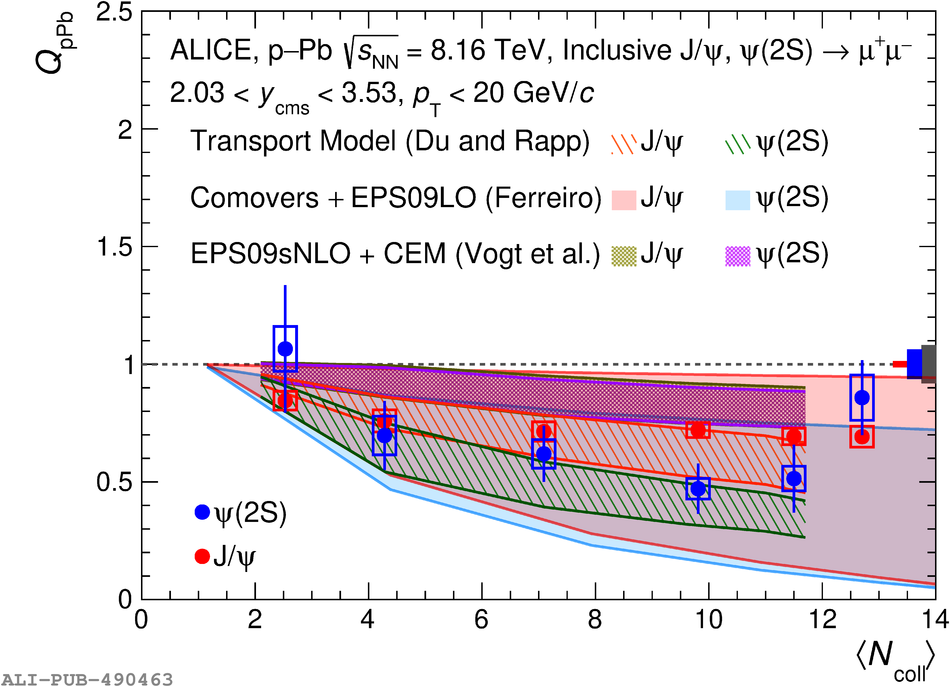# Figure 11

 Inclusive $\psi$(2S) $Q_{\rm pPb}$ as a function of $\langle N_{\rm coll} \rangle$ at backward (left) and forward (right) rapidity compared to J/$\psi$ $Q_{\rm pPb}$ and with the theoretical models. Vertical error bars represent the statistical uncertainties, while the open boxes around the data points correspond to the uncorrelated systematic uncertainties. The red and blue boxes around unity represent the correlated systematic uncertainty specific to the J/$\psi$ and $\psi$(2S), respectively. The grey box corresponds to the common systematic uncertainty correlated over $\langle N_{\rm coll}\rangle$.